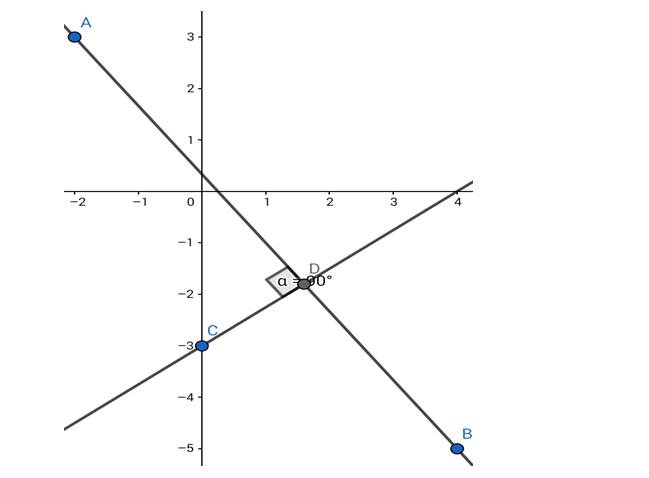# Find the equation of the line whose yQuestion:

Find the equation of the line whose y - intercept is - 3 and which is perpendicular to the line joining the points ( - 2, 3) and (4, - 5).

Solution:Given: The line perpendicular to the line passing through $(-2,3)$ and $(4,-5)$ has the $y-$ intercept of $-3$.

Formula to be used: If (a,b) and (c,d) are two points then the equation of the line passing through them is

$\frac{\mathrm{y}-\mathrm{d}}{\mathrm{x}-\mathrm{c}}=\frac{\mathrm{d}-\mathrm{b}}{\mathrm{c}-\mathrm{a}}$

Product of slopes of two perpendicular lines $=-1$

The equation of the line joining points $(-2,3)$ and $(4,-5)$ is

$\frac{y-(-5)}{x-4}=\frac{(-5)-3}{4-(-2)}$

or, $\frac{y+5}{x-4}=\frac{-8}{6}=-\frac{4}{3}$

or, $3 y+15=-4 x+16$ or, $4 x+3 y=1$

Slope of this line is $-4 / 3$.

∴ the slope of the perpendicular line =

$\frac{-1}{-4 / 3}=3 / 4$

The equation of the line can be written in the form

$y=\left(\frac{3}{4}\right) x+c$

( $c$ is the $y$ - intercept)

But, the $y$ - intercept is $-3$

The required line is

$y=\frac{3}{4} x+(-3)$

i.e. $3 x-4 y=12$